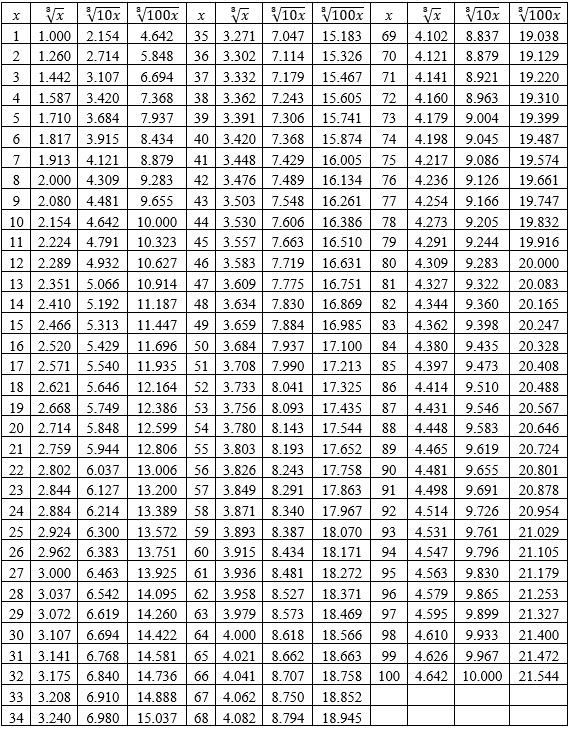Table of Cube RootsQuestion: Using cube root table, find the values of:

(i)$\sqrt{7}$     (ii)$\sqrt{30}$     (iii)$\sqrt{59 }$     (vi)$\sqrt{110}$      (v)$\sqrt{350}$

(vi)$\sqrt{4000}$     (vii)$\sqrt{490}$      (viii)$\sqrt{504}$      (ix)$\sqrt{2214}$     (x)$\sqrt{2875}$

(xi)$\sqrt{734}$    (xii)$\sqrt{914}$

Using cube root table, find the values of:

(i)$\sqrt{7}= 1.913$

(ii)$\sqrt{30} = \sqrt{(3 \times 10)}=3.170$

(iii)$\sqrt{59 }=3.893$

(vi)$\sqrt{110} = \sqrt{(11 \times 10)}=4.791$

(v)$\sqrt{350} = \sqrt{(35 \times 10)}=7.047$

(vi)$\sqrt{4000} = \sqrt{(40 \times 100)}= 15.874$

(vii)$\sqrt{490} = \sqrt{(49 \times 10)}=7.884$

(viii)$\sqrt{504} = \sqrt{(8 \times 63)} =2 \sqrt{63} =2 \times 3.979=7.958$

(ix)$\sqrt{2214} = \sqrt{(2 \times 3 \times 3 \times 3 \times 41)} = 3 \sqrt{82}=3 \times 4.344 = 13.032$

(x)$\sqrt{2875}= \sqrt{(5 \times 5 \times 5 \times 23)}=5 \sqrt{23}=5 \times 2.844=14.22$

(xi)$\sqrt{734}$$\sqrt{730} < \sqrt{734} < \sqrt{740}$$\sqrt{(73 \times 10)}< \sqrt{734}< \sqrt{(74 \times 10)}$$9.004< \sqrt{734}< 9.045$

For a difference of$10$, the difference in values$= 9.045 - 9.004 = 0.041$

For a difference of$4$, the difference in value$\displaystyle = \frac{(0.041 \times 4) }{ 10} = 0.0164$

Therefore$\sqrt{734}=9.004+0.0164=9.0204$

(xii)$\sqrt{914}$$\sqrt{910}< \sqrt{914}< \sqrt{920}$$\sqrt{(91 \times 10)}< \sqrt{914}< \sqrt{(92 \times 10)}$$9.691< \sqrt{914}< 9.726$

For a difference of$10$, the difference in values$= 9.726-9.691=0.035$

For a difference of$4$, the difference in value$\displaystyle = \frac{(0.035 \times 4) }{ 10} = 0.014$

Therefore$\sqrt{914}=9.691+0.014=9.705$